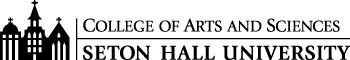# B.S. Financial Mathematics/M.S. Financial Engineering Curriculum

#### Freshman Year

CSAS 1114 Introduction to Program Design I 3
MATH 1501 Calculus I for Mathematics & Physical Sciences I 4
MATH 1511 Calculus for the Mathematical and Physical Sciences II 4
MATH 1611 Introductory Discrete Mathematics 3
PHIL 1204 Symbolic Logic* (recommended) 3
ECON 1402 Principles of Economics 3
ECON 1403 Principles of Economics II 3

#### Sophomore Year

MATH 2511 Calculus for the Mathematical and Physical Sciences III 4
MATH 2711 Introduction to Probability and Statistics 4
MATH 2813 Linear Algebra 4
BACC 2103 Financial Accounting 3

#### Junior-Senior Year

MATH 3515 Analysis 4
MATH3711 Statistical Analysis I 3
MATH 6721 Financial Calculus I 3
MATH 7722 Adv. Topics in Financial Calculus 3
BFIN 4 of the following 5 courses:
BFIN 3211 Financial Strategy 4
BFIN 4227 Investment Analysis 3
BFIN 4250 Fixed Income Analysis 3
BFIN 4253 Advanced Corporate Finance 3
BFIN 4255 Financial Modeling 3

#### Junior-Senior Year Courses Taken Towards the M.S. in Financial Engineering

• Take the graduate course DAVA 7000 Data Visualization which will count towards FE550 Data Visualizations Applications in the M.S. in Financial Engineering.
• Take the graduate course MATH 6721 Financial Calculus I (required for B.S. in Financial Mathematics) that counts towards FE530 Introduction to Financial Engineering for the M.S. in Financial Engineering.
• Take the graduate course MATH 7722 Financial Calculus II (required for B.S. in Financial Mathematics) which counts towards FE543 Introduction to Stochastic Calculus for Finance for the M.S. in Financial Engineering as well as the B.S. in Financial Mathematics.

#### Last Year as Graduate in M.S. in Financial Engineering

Core Courses
FE610 Stochastic Calculus for Financial Engineers
FE620 Pricing and Hedging
FE621 Computational Methods in Finance
FE630 Portfolio Theory and Applications
FE800 Project in Financial Engineering

Elective Courses
FE530 Introduction to Financial Engineering (Credit from Seton Hall MATH 6721)
FE535 Introduction to Financial Risk Management
FE540 Probability theory for Financial Engineering
FE541 Applied Statistics with Applications in Finance
FE542 Time Series with Applications to Finance
FE543 Introduction to Stochastic Calculus for Finance (Credit from Seton Hall MATH 7722)
FE545 Design, Patterns and Derivatives Pricing
FE550 Data Visualization Applications (Credit from Seton Hall DAVA 7000)
FE555 2D Data Visualization Programming for Financial Applications
FE570 Market Microstructure and Trading Strategies
FE571 Quantitative Hedge Fund Strategies
FE575 Introduction to Econophysics
FE580 Securitization of Financial Assets
FE582 Foundations of Financial Data Science
FE590 Statistical Learning in Finance
FE595 Financial Technology (FinTech)
FE622 Simulation Methods in Computational Finance and Economics
FE625 Emerging Markets: Risks and Models
FE635 Financial Enterprise Risk Engineering
FE641 Multivariate Statistics and Advanced Time Series in Finance
FE655 Systemic Risk and Financial Regulation